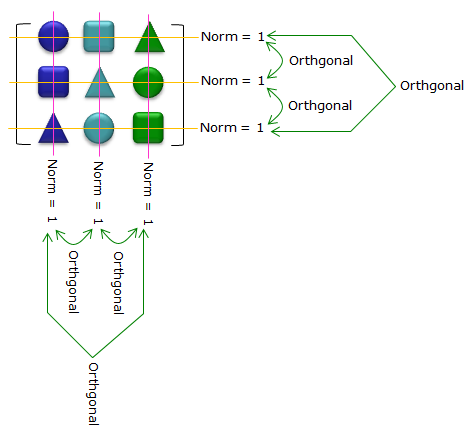Engineering Math - Quick Reference                                 Home : www.sharetechnote.com Matrix-Unitary Matrix   Unitary Matrix is a special kind of complex square matrix which has following properties. (U in the following description represents a unitary matrix) U*U = UU* = I (U* is the conjugate transpose of the matrix U) |det(U)| = 1 (It means that this matrix does not have scaling properties, but it can have rotating property) Eigenspaces of U are orthogonal U is diagonalizable U* is unitary U is invertible and the inverse of U is U* The columns of U forms an orthnomal basis The rows of U forms an orthnomal basis The eigenvalues of U lies on the unit circle and they are normal to each other